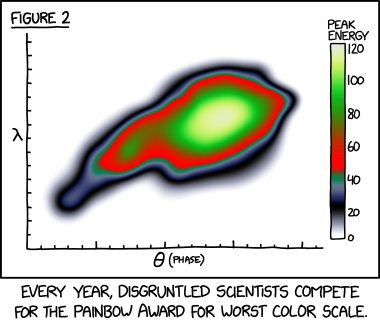# Painbow

Painbow lets you use XKCD’s “painbow” colormap in ggplot.

XKCD implied that this colormap is terrible, and even called it a “painbow”. However, these examples show that with certain tasks and data, this colormap outperforms even some of the most commonly cited “good” colormaps like viridis.Here’s a reproduction in ggplot using some custom theming:## Installation

You can install the latest development version:

``````install.packages("devtools")
devtools::install_github("steveharoz/painbow")``````

## Examples

Setup:

``````library(tidyverse)
library(painbow)
library(patchwork) # combine multiple graphs``````

### A simple example for the scale:

``````ggplot(faithfuld) +
aes(waiting, eruptions, fill = density) +
geom_raster(interpolate = TRUE) +
scale_fill_painbow() +
labs(title = "Can you find the most dense region?") +
theme_bw(18)``````### The dataset from the comic

The dataset is `painbow_data`. It was made using the comic’s image and a scripted lookup table.Here is a 2D field with a regular pattern and a deviation. Can you find it? Painbow makes a task easier compared with commonly touted “good” colormaps.

``````##### 2D #####

COUNT = 512

data = expand.grid(
x = 1:COUNT,
y = 1:COUNT) %>%
mutate(z = sin(x/16) + cos(y/16)) %>%
mutate(znoise = z + dnorm(sqrt((x-0.75*COUNT)^2 + (y-0.33*COUNT)^2)/COUNT*20))

ggplot(data) +
aes(x=x, y=y, fill=znoise) +
geom_raster() +
labs(title = "ggplot default", fill=NULL) +
theme_void(15) + theme(legend.text = element_blank()) +
ggplot(data) +
aes(x=x, y=y, fill=znoise) +
geom_raster() +
scale_fill_viridis_c() +
labs(title = "Viridis", fill=NULL) +
theme_void(15) + theme(legend.text = element_blank()) +
ggplot(data) +
aes(x=x, y=y, fill=znoise) +
geom_raster() +
scale_fill_painbow() +
labs(title = "XKCD's colormap", fill=NULL) +
theme_void(15) + theme(legend.text = element_blank()) +
patchwork::plot_annotation(
title = "Three colormaps. Same data. Can you spot the weird region?",
theme = theme(text = element_text(size=20)))``````### Painbow can help you spot a subtle pattern among data with high dynamic range

``````######## 1D #########

COUNT = 1024

data = tibble(
x = 1:COUNT,
y = x/COUNT + sin(x/4)/100
)

ggplot(data) +
aes(x = x, y=x) +
geom_line() +
labs(title = "y = x") +
theme_void(15) + theme(legend.text = element_blank()) +
ggplot(data) +
aes(x = x, y=COUNT/2, fill=x) +
geom_tile(width=1, height=COUNT, color=NA) +
labs(title = "ggplot default") +
theme_void(15) + theme(legend.text = element_blank()) +
ggplot(data) +
aes(x = x, y=COUNT/2, fill=x) +
geom_tile(width=1, height=COUNT, color=NA) +
scale_fill_viridis_c() +
labs(title = "viridis") +
theme_void(15) + theme(legend.text = element_blank()) +
ggplot(data) +
aes(x = x, y=COUNT/2, fill=x) +
geom_tile(width=1, height=COUNT, color=NA) +
scale_fill_painbow() +
labs(title = "painbow") +
theme_void(15) + theme(legend.text = element_blank()) +

ggplot(data) +
aes(x = x, y=y) +
geom_line() +
labs(title = "y = x + sine wave") +
theme_void(15) + theme(legend.text = element_blank()) +
ggplot(data) +
aes(x = x, y=COUNT/2, fill=y) +
geom_tile(width=1, height=COUNT, color=NA) +
labs(title = "ggplot default") +
theme_void(15) + theme(legend.text = element_blank()) +
ggplot(data) +
aes(x = x, y=COUNT/2, fill=y) +
geom_tile(width=1, height=COUNT, color=NA) +
scale_fill_viridis_c() +
labs(title = "viridis") +
theme_void(15) + theme(legend.text = element_blank()) +
ggplot(data) +
aes(x = x, y=COUNT/2, fill=y) +
geom_tile(width=1, height=COUNT, color=NA) +
scale_fill_painbow() +
labs(title = "painbow") +
theme_void(15) + theme(legend.text = element_blank()) +

patchwork::plot_layout(ncol=4) +
patchwork::plot_annotation(
title = "Three colormaps. Same data. Can you spot the harmonic?",
theme = theme(text = element_text(size=20)))``````## Feedback, issues, and contributions

Feedback, suggestions, issues, and contributions are all welcome! Please file an issue or pull request at https://github.com/steveharoz/painbow/issues

## Citing Painbow

The XKCD comic deserves credit: https://xkcd.com/2537/